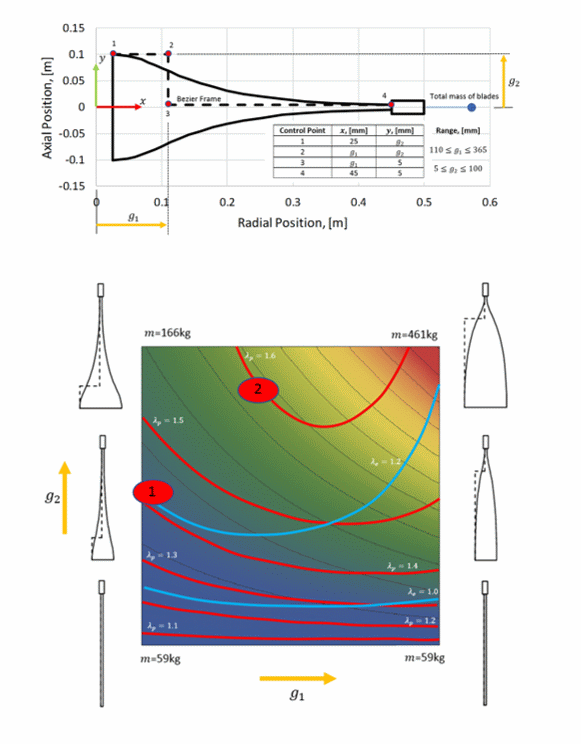# Lamé Finite Element (LFE)

The Lamé Finite Element (LFE) is an axisymmetric membrane element formulated directly from stress fields satisfying the Lamé equations. As such it is an Equilibrium Finite Element (EFE) and exhibits the useful properties of elements based on this formulation. The element can be loaded with boundary forces and displacements as well as body loads due to rotation and temperature variation. Plasticity is included so that, for example, both elastic and plastic limit loads (pressures for pipes and speeds for rotating discs) can be calculated.

### LFE Code Verification